×#### Thank you for registering.

One of our academic counsellors will contact you within 1 working day.

Click to Chat

1800-1023-196

+91-120-4616500

CART 0

• 0

MY CART (5)

Use Coupon: CART20 and get 20% off on all online Study Material

ITEM
DETAILS
MRP
DISCOUNT
FINAL PRICE
Total Price: Rs.

There are no items in this cart.
Continue Shopping```Equal Sets

Table of Content

Meaning of Sets

What are the Elements of a Set?

Definition of Equal Sets

Equal Sets in Math

Cardinality of Equal Sets

Symbol of Equal Sets

What is the definition of Equivalent Sets in math?

Symbol of Equivalent Sets

What is the difference between Equal and Equivalent Sets?

Relation between Equal and Equivalent Sets

How to determine sets as Equal or Equivalent Sets?

What is the difference between Joint and Disjoint Sets?

Graphical Representation of Joint and Disjoint Sets

Meaning of Sets

A collection of different objects having some common property is called a Set. It could be anything like numbers, pictures alphabets etc. In a set we have to list all the objects of the group then enclose them in the “{ }”curly braces.

What are the Elements of a Set?

The different objects of a set are called the elements of a set. The elements and members are one and a same thing. Generally, we denote a set with capital letters. We have to list all the objects and separate them with the comma and then enclose them in the curly braces.

Example 1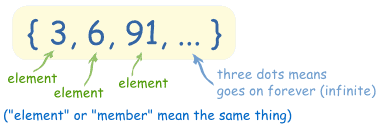In the above picture 3, 6 and 91 are the elements of a set so we have to write them in the brackets. Here the three dots represent that the no. of elements are unlimited.

Example 2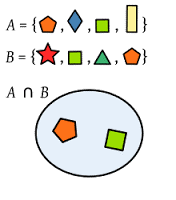Here the shapes are the elements of the set and the set is denoted by capital letter “B”. And the number of elements of set B is 4 here. We represent it as n(B) = 4.

Definition of Equal Sets

The two given sets are called Equal Sets if their number of elements and the member of elements are exactly same. It could be anything like number, pictures and alphabets etc.The order of elements and the repetition of elements does not have any relevance here.

Example 1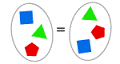Here, these are the two sets of shapes.These are equal sets because there number of elements are same and there elements are also same.

Example 2

A = {5, 6, 7, 8}

B = {6, 8, 5, 7}

C = {5, 5, 6, 6, 7, 7, 8, 8}

Here all the three sets, set A, set B and set C are equal, as their elements are same irrelevance of order and the repetition.

Equal Sets in Math

The two sets X and Y are called equal sets if all the elements of X are present in Y and all the elements of Y are present in X. Or you can say that if both the sets are the subset of each other then they are equal sets.

X⊂Y and Y⊂X ⇔X=Y

X is the subset of Y and Y is the subset of X, it implies that X is equal to Y.

Example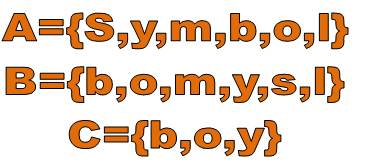Here all the elements of A and B are same,or we can say that A is the subset of B and B is a subset of A, So A=B

But if this condition does not fulfill then the sets are called unequal sets.

As in the above example,

C⊂A but A⊄C ⇔ A≠C

That is, all the elements of C are present in A but all the elements of A are not present in C, it implies that A is not equal to C or A and C are unequal sets.

So, if all the elements of two given sets are not same then these are called Unequal Sets.

Cardinality of Equal Sets

The cardinality of a set is n(A)= x, where, x represents the number of elements of set A.

As in the above picture , the cardinality of this set A is 6 and set B is also 6.

n(A) = n(B) = 6

So according to the number of elements or in cardinal terms , two sets are called equal sets if ,

n(A) = n(B) and x∈A, x∈B also,

Then,

A = B

Symbol of Equal Sets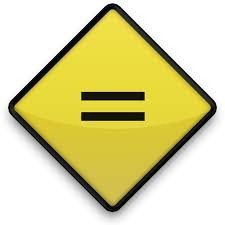To represent the equal sets we use a symbol of  “=” i.e. equality.

And for unequal sets we use the symbol of “≠” i.e. not equal to.

As in the above example,

A = B i.e. Set A is equal to Set B

But, A ≠ C i.e. Set A is not equal to Set C.

What is the definition of Equivalent Sets in math?

Two sets are called Equivalent Sets if there number of elements are same .The elements need not to be same, just the number of elements should be same.Here, all the four sets are equivalent sets as the number of elements are same in all the four sets of triangle, smiley, star and heart Here n(T) = n(Sm) = n(St) = -n(H) = 6.

So sets are said to be equivalent if there cadinality is same.

As all the empty sets has zero cardinality, so all the empty sets are equivalent sets.

Another definition of equivalent sets is that two sets are equivalent if there is bijection within two sets.The bijection is the one to one correspondence between two sets.It means that for every elemnet in Set X there must be an Element in Set Y, till the elements get over.

Example

A Bijection is a One – To – One Correspondence Between Sets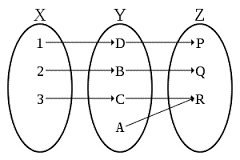Here in the above picture X and Z are equivalent sets as there elements are not same but the number of elements is same. For every element In Set X there is an element in set Z also. But set X and Y are not equivalent because for every element in Y there is no element in X.There are 3 elements in X and 4 elements in Y.

So two sets are equivalent if there is bijection within them.

Symbol of Equivalent Sets

To represent equivalent sets we use equivalent sign  or “ ~” or “≡”

As in the above example X ~ Z i.e. Set X is equivalent to Set Z.

What is the diference between Equal and Equivalent Sets?

The difference between Equal and Equivalent Sets is as follows:

No.
Points
Equal sets
Equivalent sets

1

Definition

Two sets are equal if all the elements of both the sets are same.

Two sets are equivalent if the number of elements of both the sets is same.

2

Number of elements

Same in both sets

Same in both sets

3

Cardinality

Same of both sets

Same of both sets

5

Elements

Elements should be same

Elements need not be same

6

Symbol

=

~ or ≡

7

Relation

Equal sets can be equivalent also.

Equivalent sets cannot be equal.

8

Example

A={2,4,6,8}

B={4,8,2,6}

A=B

X= {2, 4, 6, 8}

Y={1,3,5,7}

X~Y

Relation between Equal and Equivalent Sets

Two sets are equal if they are exactly same i.e. their elements and the number of elements both are same without any relevance of order and repetition of elements.

And two sets are equivalent if their number of elements are same. There elements could be same or different, just the number should be same.

Equal sets are equivalent also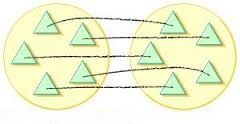Here in the above picture both the sets are equal because there all the elements are same and as there number of elements are also same so these are equivalent also.

So all equal sets are equivalent also.

Equivalent sets may not be equal sets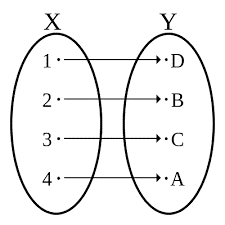Here X and Y are equivalent sets as their number of elements are same i.e. 4, but these are not equal sets because there elements are not same.

So all equivalent sets are not equal sets.

How to determine sets as Equal or Equivalent Sets?

As we know that all the sets which have exact same element and same number of element are called equal sets and the sets having just same number of elements are equivalent set. So it is easy to identify the sets as equal or equivalent sets.

Let’s try some examples to determine the equal and equivalent sets.

S.Np.
Example
Equal or Equivalent
Why?

1

A = {5,10,15,20}

Equal and equivalent both

Both the sets have exact same elements and the order of elements has no relevance.

B = {10,5,20,15}

2

C = {5, 10, 15, 20}

Equivalent but not equal

Both the sets have same number of elements but the elements are different.

D = {@,\$,&,#}

3

E = {1,2,3,4,5}

Equal  and equivalent both

Both the sets have same elements and the repetition of elements does not have any relevance.

F = {1,1,2,2,3,3,4,4,5,5}

4

G = {1,2,3,4}

Not equal nor equivalent

Both the sets have different number of elements and the elements also are not exactly same.

H = {1,2,3,4,5}

5

I = {A,B,C,D}

Equivalent but not equal

The number of elements is same in both the sets.

J =Sun,Mon,Tue,Wed}

6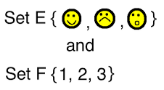Equivalent but not equal

The number of elements is same in both the sets.

This shows that all the equal sets are equivalent also but all equivalent sets are not equal.

What is the difference between Joint and Disjoint Sets?

Joint Sets

Two sets are said to be joint if there is at least one element common within them.

Symbolically,

A∩B ≠ ∅ i.e. A intersection B is not an empty set.

Example

A = {1, 2, 3, 4}

B = {2, 4, 6, 8}

A∩B = {2,4} ≠ ∅

Here A and B are joint sets because there are two elements common in A and B.

Disjoint Sets

Two sets are said to be disjoint if there is no common element within them.

Symbolically,

A∩B = ∅ i.e. A intersection B is an empty set.

Example

A= {1, 2, 3, 4}

B= {5, 6, 7, 8}

A∩B = ∅

Here A and B are disjoint sets because there is no common element within them.

Graphical Representation of Joint and Disjoint Sets

The joint and disjoint sets can be represented with Venn diagram. It is the easy way to understand the concept of joint and disjoint sets.

If the two sets are represented by two mutually exclusive circles and they are not intersecting with each-other, then these sets are called disjoint sets. As the name represents itself that the sets are not joining with each other.

If two sets are represented by two circles which are intersecting with each-other, then these are the joint sets. This shows that there is some common element within them which is shown by the intersection part of the two circles.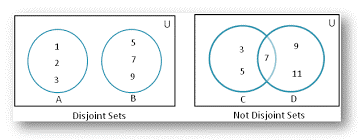Here set A and set B are disjoint sets as they have no common element within them. They are mutually exclusive.

A = {1, 2, 3}

B = {5, 7, 9}

When two sets are disjoint then their intersection is empty.

A∩B =∅

Set C and D are joint sets as they have one element common within them.

C = {3, 5, 7}

D = {7, 9, 11}

C∩D = {7}

Equal Sets
```### Course Features

• 731 Video Lectures
• Revision Notes
• Previous Year Papers
• Mind Map
• Study Planner
• NCERT Solutions
• Discussion Forum
• Test paper with Video Solution